• ## RNN和LSTM的参数计算

千次阅读 2019-04-17 11:55:08
RNN（Recurrent Neural Network）是一类用于处理序列数据神经网络。神经网络包含输入层、隐含层、输出层，通过激活函数控制输出，层与层之间通过...这里主要写一下RNN中参数的计算： 再加上偏执值b，即可得到...
RNN（Recurrent Neural Network）是一类用于处理序列数据的神经网络。神经网络包含输入层、隐含层、输出层，通过激活函数控制输出，层与层之间通过权值连接，在标准的RNN结构中，隐层的神经元之间也是带有权值的。也就是说，随着序列的不断推进，前面的隐层将会影响后面的隐层。
具体结构如下图，本文不再赘述。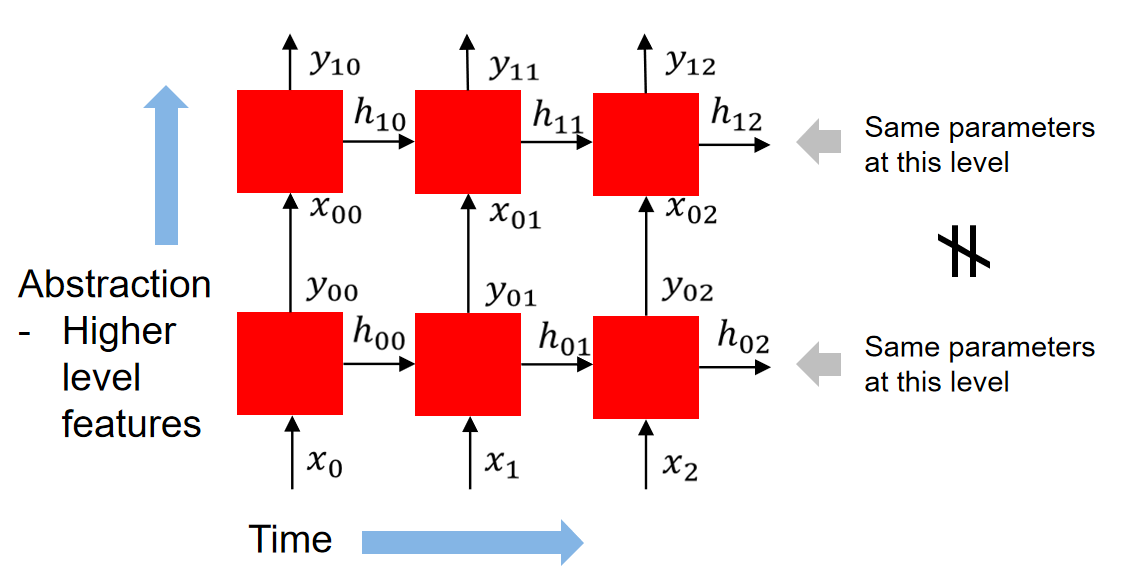这里主要写一下RNN中参数的计算：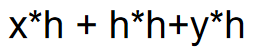再加上偏执值b，即可得到模型中参数总和。其中，h为隐含层单元，x为输入，y为输出。
例如，在手写字体识别中，用64层的隐含层网络（h），28维的输入（x），10个分类的输出（y），这里的参数总和就是：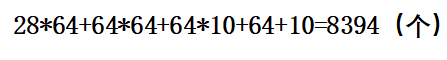最后加64加10即为隐含层和输出层的偏执值。
LSTM
LSTM是对RNN模型长期依赖的改进，通过三个门结构来实现信息的保护和控制。这三个门分别为输入门、遗忘门和输出门。
所以LSTM隐藏层参数是RNN的四倍，所以此LSTM网络的参数个数为：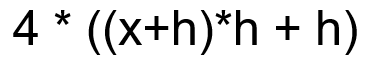同样再加上偏执值。即为参数的总值。理解LSTM的原理就不难理解这个公式。
同样，在手写字体识别中，用64层的隐含层网络（h），28维的输入（x），10个分类的输出（y），这里的参数总和就是：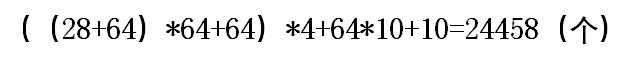以上即为RNN和LSTM的参数总和计算。


展开全文• 本文就来补充一下，讲讲如何计算 LSTM 的参数数量。 建议阅读本文前先阅读 Understanding LSTM Networks 的原文或我的译文。 首先来回顾下 LSTM。一层 LSTM 如下： 这里的 xtx_txt​ 实际上是一个句子的 ...
理论上的参数量
之前翻译了 Christopher Olah 的那篇著名的 Understanding LSTM Networks，这篇文章对于整体理解 LSTM 很有帮助，但是在理解 LSTM 的参数数量这种细节方面，略有不足。本文就来补充一下，讲讲如何计算 LSTM 的参数数量。

建议阅读本文前先阅读 Understanding LSTM Networks 的原文或我的译文。

首先来回顾下 LSTM。一层 LSTM 如下：这里的 $x_t$ 实际上是一个句子的 embedding（不考虑 batch 维度），shape 一般为 [seq_length, embedding_size]。图中的 $A$ 就是 cell，$x_t$ 中的词依次进入这个 cell 中进行处理。可以看到其实只有这么一个 cell，所以每次词进去处理的时候，权重是共享的，将这个过程平铺展开，就是下面这张图了：实际上我觉得这里 $x_t$ 并不准确，第一个 $x_t$ 应该指的是整句话，而第二个 $x_t$ 应该指的是这句话中最后一个词，所以为了避免歧义，我认为可以将第一个 $x_t$ 重命名为 $x$，第二个仍然保留，即现在 $x$ 表示一句话，该句话有 $t+1$ 个词，$x_t$ 表示该句话的第 $t+1$ 个词，$t \in [0, t]$。

始终要记住这么多 $A$ 都是一样的，权重是一样的，$x_0$ 到 $x_t$ 是一个个词，每一次的处理都依赖前一个词的处理结果，这也是 RNN 系的网络难以像 CNN 一样并行加速的原因。同时， 这就像一个递归过程，如果把求 $h_t$ 的公式展开写，$A$ 里的权重记为 $W$，那么就会发现需要 $t$ 个 $W$ 相乘，即 $W^t$，这是非常恐怖的：
$0.9^{100} = 2.6561398887587544 \times 10^{-5}$
$1.1^{100} = 13780.61233982238$
一个不那么小的数被多次相乘之后会变得很小，一个不那么大的数被多次相乘之后会变得很大。所以，这也是普通 RNN 容易出现梯度消失/爆炸的问题的原因。
扯远了点。
那么 LSTM 的参数很明显了，就是这个 $A$ 中的参数。这个 $A$ 内部具体是这样的：
[外链图片转存失败,源站可能有防盗链机制,建议将图片保存下来直接上传(img-KODbF2C1-1603458457529)(https://i.loli.net/2020/06/03/3UDWxmOfbr7acE4.png)]
从这张图来理解参数的数量你可能有点懵逼，一步一步来看，实际上这里面有 4 个非线性变换（3 个 门 + 1 个 tanh），每一个非线性变换说白了就是一个两层的全连接网络。重点来了，第一层是 $x_i$ 和 $h_i$ 的结合，维度就是 embedding_size + hidden_size，第二层就是输出层，维度为 hidden_size，所以该网络的参数量就是：
(embedding_size + hidden_size) * hidden_size + hidden_size

一个 cell 有 4 个这样结构相同的网络，那么一个 cell 的总参数量就是直接 × 4：
((embedding_size + hidden_size) * hidden_size + hidden_size) * 4

注意这 4 个权重可不是共享的，都是独立的网络。
所以，一般来说，一层 LSTM 的参数量计算公式是：
$4[d_h(d_h + d_x) + d_h]$
其中 4 表示有 4 个非线性映射层，$d\_h + d\_x$ 即 Understanding LSTM Networks 中的 $[h\_{t-1}, x\_t]$ 的维度，后面的 $d_h$ 表示 bias 的数量。所以，LSTM 层的参数数量只与输入维度 $d\_x$ 和输出维度 $d\_h$ 相关，和普通全连接层相同。
那么显而易见，一层双向 LSTM 的参数量就是上述公式 × 2。
TensorFlow 中的实现
在 TensorFlow 中，这些 $d_x$、$d_h$ 如何与代码对应上呢？
我们可以如下实现一个简单的以 LSTM 为核心的网络：
import tensorflow as tf

model = tf.keras.model.Sequential(
tf.keras.layers.Embedding(1000, 128),
tf.keras.layers.LSTM(units=64),
tf.keras.layers.Dense(10)
)
model.summary()

输入如下：
Model: "sequential"
_________________________________________________________________
Layer (type)                 Output Shape              Param #
=================================================================
embedding_1 (Embedding)      (None, None, 128)         128000
_________________________________________________________________
lstm_1 (LSTM)                (None, 64)                49408
_________________________________________________________________
dense_1 (Dense)              (None, 10)                650
=================================================================
Total params: 178,058
Trainable params: 178,058
Non-trainable params: 0
_________________________________________________________________

可以看到 TF 给出的 LSTM 层参数量是 49408。我们来根据上面的公式验证下。

$d_x$：输入维度，在这里就对应于 128，就是词向量维度。
$d_h$：输出维度，在这里就是 LSTM 的参数 64，在 TF 这里叫 units。

所以，参数量就是 $4 \times \left[64 \times \left(64+128\right) + 64 \right] = 49408$，和 TF 给出的一样。
另外，tf.keras.layers.LSTM() 的默认输出大小为 [batch_size, units]，就是只使用最后一个 time step 的输出。假如我们想要得到每个 time step 的输出（$h_0,\cdots,h_t$）和最终的 cell state（$C_t$），那么我们可以指定另外两个参数 return_sequences=True 和 return_state=True：
inputs = tf.random.normal([64, 100, 128])  # [batch_size, seq_length, embedding_size]
whole_seq_output, final_memory_state, final_carry_state = tf.keras.layers.LSTM(64, return_sequences=True, return_state=True)(inputs)
print(f"{whole_seq_output.shape=}")
print(f"{final_memory_state.shape=}")
print(f"{final_carry_state.shape=}")

输出：
whole_seq_output.shape=TensorShape([32, 100, 64])  # 100 表示有 100 个词，即 100 个 time step
final_memory_state.shape=TensorShape([32, 64])
final_carry_state.shape=TensorShape([32, 64])

OK，LSTM 的参数量应该挺清晰了，欢迎在评论区留下你的想法。😋
Reference

Counting No. of Parameters in Deep Learning Models by Hand
deep learning - Number of parameters in an LSTM model - Data Science Stack Exchange
machine learning - How to calculate the number of parameters of an LSTM network? - Stack Overflow
tensorflow - In Keras, what exactly am I configuring when I create a stateful LSTM layer with N units? - Stack Overflow
理解 LSTM 网络 · Alan Lee
Recurrent Neural Networks (RNN) with Keras  |  TensorFlow Core
LSTM is dead. Long Live Transformers! - YouTube

END


展开全文1024程序员节 自然语言处理 tensorflow
• 参数计算1 首先这篇博客不是介绍LSTM原理的，因为我也不敢说我已经完全理解了LSTM。。。。。。好了，言归正传，我们开始计算lstm的参数，首先来看pytorch中公式怎么写的： it=σ(Wiixt+bii+Whih(t−1)+bhi)ft=σ...
参数计算

首先这篇博客不是介绍LSTM原理的，因为我也不敢说我已经完全理解了LSTM。。。。。。好了，言归正传，我们开始计算lstm中的参数，首先来看pytorch中公式怎么写的：
$\begin{array}{ll} \\ & \bm{i_t = \sigma(W_{ii} x_t + b_{ii} + W_{hi} h_{t-1} + b_{hi}) } && (1)\\\\ & \bm{f_t = \sigma(W_{if} x_t + b_{if} + W_{hf} h_{t-1} + b_{hf}) }&& (2)\\\\ & \bm{g_t = \tanh(W_{ig} x_t + b_{ig} + W_{hg} h_{t-1} + b_{hg})} && (3)\\\\ & \bm{o_t = \sigma(W_{io} x_t + b_{io} + W_{ho} h_{t-1} + b_{ho}) }&& (4)\\\\ & \bm{c_t = f_t * c_{t-1} + i_t * g_t} && (5)\\\\ & \bm{h_t = o_t * \tanh(c_t)} && (6)\\ \end{array}$
这里需要注意的是pytorch没有采用拼接的方法将x 和 h进行拼接，而是分开计算，同时每个公式多了一个偏置。 当然,这对LSTM的理解无关紧要了，但是在计算参数数量上是有差别的。这里我们假设input_size=100, hidden_size=100 代码如下：
rnn=nn.LSTM(input_size=100,hidden_size=100,num_layers=1,bidirectional=False)
for p in rnn.parameters():
print(p.size())

output：
torch.Size([400, 100])
torch.Size([400, 100])
torch.Size()
torch.Size()

接下来我们来看这个参数是怎么计算来的。首先我们要明确一点就是我们要计算的参数是什么，我们要计算的参数是 w,b。我们知道LSTM中有三个门控制单元和一个输入单元，他们的的参数大小是一样的，所以只需要计算一个然后乘以4就行了。如果对LSTM不熟悉也可以从上面的公式里看出一共8对$w,b$.首先我们知道 $x$ 是一个100维的向量，看公式：(1) 我们知道经过 $sigmoid$ 之后应该也是100维的向量那么从而可以推断出 $W x$ 也是100维喽。那么我们就可以得到：
$\bm{W[100*100]*x[100*1]=[100*1]\tag7}$
这样就得到了 $W$ 大小啦 $b$  的大小同样可以推断出 $b$ 是100维啦。然而会有人疑问输出明明是 [400 *  100] 而不是[100 * 100]我们观察上面LSTM的前四个公式是不是一共八个 $W$ ，左边四个，右边四个，嗯，没错 [400 * 100] 就是分别代表左边四个 $W$ 的总大小，当然，也就是对应的b的大小了。这里一共 40000+40000+800=80800 个参数

注意 1

另外说一点就是其他的博客有通过公式：$4(mn+n^2+n)$ 来计算总参数，其中 m 是 input_size，n 是 hidden_size.对应我们上面例子就是 m=n=100 然后计算出 80400 个参数。为什么和上面的不一样呢。其实是一样的，我在开头也说了，pytorch 没有采用拼接的方法，并且每个公式多处一个偏置也就是一共多出 4 个。一个 100 维度也就是 400 个参数。嗯，到这里计算结束了。

注意 2

当网络是双层或者双向时，只需要把参数乘以2即可，但是这里要注意的是，当网络为双层双向时就需要注意了。废话不多说先看代码效果好吧，代码如下：
rnn=nn.LSTM(input_size=100,hidden_size=100,num_layers=2,bidirectional=True)
for p in rnn.parameters():
print(p.size())
output:
torch.Size([400, 100])
torch.Size([400, 100])
torch.Size()
torch.Size()
torch.Size([400, 100])
torch.Size([400, 100])
torch.Size()
torch.Size()
torch.Size([400, 200])
torch.Size([400, 100])
torch.Size()
torch.Size()
torch.Size([400, 200])
torch.Size([400, 100])
torch.Size()
torch.Size()

不知道大家注意到了没有倒数第四行和倒数第八行是[400 * 200],为什么会这样呢? 接下来，我先从pytorch给出的公式来解释，我们在计算第二层的时候其实输入x已经变了，变成多少维呢，没错是200维，forward+backward 这样，我上面说了pytorch没有采用$x ,h$拼接的方式计算而是采用分开计算，那么先来看$x$喽，我们已经知道x的维度维200 * 1，而我们想得到的输出是100那么我们就需要一个满足这个等式：
$\bm{W_i[100 * 200] * x [200 * 1] = [100 * 1]\tag8}$
我们可以看出 $W_i$ 是[100 * 200]。好了，接着我们来看 $h，h$ 还是100维的这个是hidden_size决定的。同样的我们计算出符合等式：
$\bm{W_h[100 * 100] * h [100 * 1] = [100 * 1]\tag9}$
这样 $W_h$ 也就出来了为[100 * 100]
好了分析了半天，计算总数吧，左边的 $W$ 也就是 $W_i$ 为[100 * 200] * 4 =80000 再加上$b$ * 4=400,嗯，左边一共是80400个参数，右边是 [100 * 100]* 4 +  * 4=40400.左边和右边加起来就是 120800 个参数。我们来用公式检验一下：m=200，n=100， (200 * 100+100 * 100+100)*4=120400, 至于差的400和上面的解释一样是差在 $b$ 上。

输出分析

看完下面的分析会对上面的理解更加清晰：
首先，看看输入输出的数据的格式
input: (seq_len,batch_size,dim)
h0/c0: (num_layers * num_directions, batch, hidden_size)
这个是数据的输入的格式：默认 batch_first=False;
网络的输出和输入大小差不多（主要是output的差别）；
输出是：
output：(seq_len, batch, num_directions * hidden_size)
hn/cn：(num_layers * num_directions, batch, hidden_size)
这里解释一下：
output：保存每个batch最后一层的各个time_step的输出；
hn：保存的是每个batch的每一层的最后一个time_step的输出；
cn和hn类似只是保存的是c值。
看下图会更清楚：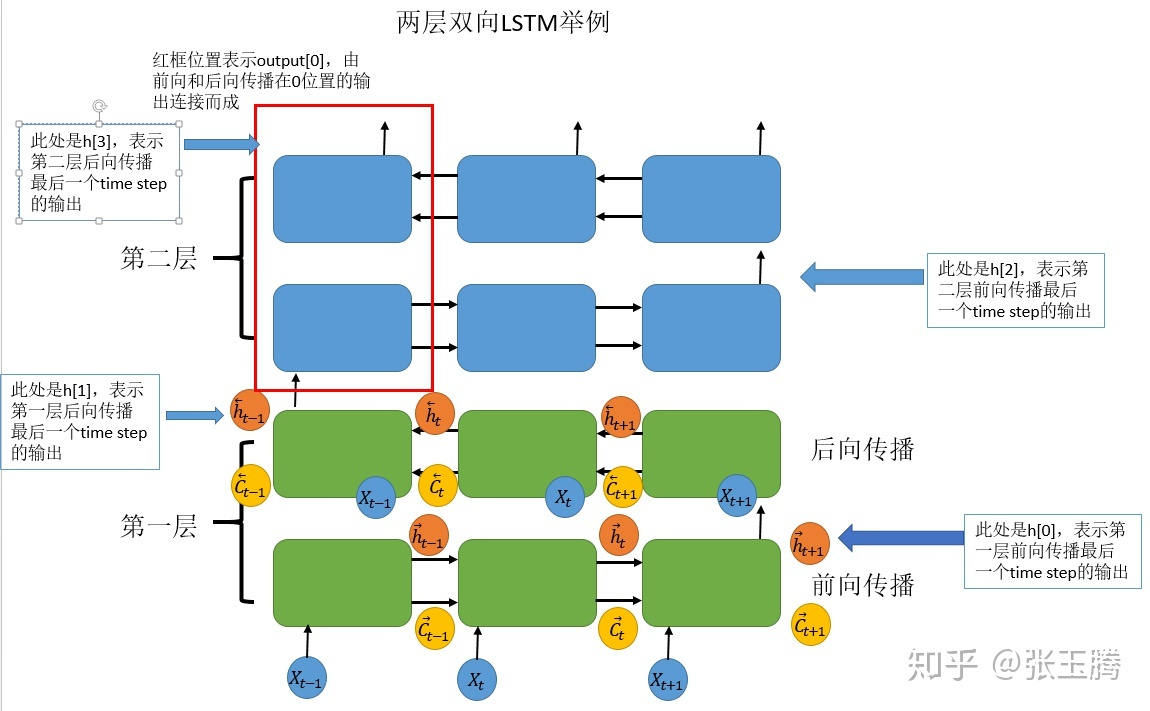参看下面程序：
import torch
import torch.nn as nn
bilstm = nn.LSTM(input_size=10, hidden_size=20, num_layers=2, bidirectional=True,batch_first=True)
'''batch:5 seq_len:3 dim:10 '''
input = torch.randn(5, 3, 10)
'''(num_layers * num_directions, batch, hidden_size)'''
h0 = torch.randn(4, 5, 20)
'''(num_layers * num_directions, batch, hidden_size)'''
c0 = torch.randn(4, 5, 20)
output, (hn, cn) = bilstm(input, (h0, c0))
print('output shape: ', output.shape)
print('hn shape: ', hn.shape)
print('cn shape: ', cn.shape)

print(output[4, 2, :20])
print(output[4, 0, 20:])
print(hn[2, 4,:])
print(hn[3, 4,:])
output:
output shape:  torch.Size([5, 3, 40])
hn shape:  torch.Size([4, 5, 20])
cn shape:  torch.Size([4, 5, 20])
tensor([-0.0395, -0.2433, -0.0611,  0.0162, -0.1448, -0.0356, -0.0575,  0.0237,
0.0697, -0.0294, -0.0441, -0.0093,  0.0053,  0.0195,  0.1096, -0.0940,
0.0843, -0.0435,  0.0702, -0.0798], grad_fn=<SliceBackward>)
tensor([ 0.0555, -0.1071,  0.0042, -0.0060, -0.0502,  0.0296,  0.1325, -0.3112,
0.1473, -0.1250,  0.0804, -0.0464,  0.0957,  0.1257,  0.0913,  0.0027,
-0.0442,  0.1325, -0.2190, -0.0221], grad_fn=<SliceBackward>)
tensor([-0.0395, -0.2433, -0.0611,  0.0162, -0.1448, -0.0356, -0.0575,  0.0237,
0.0697, -0.0294, -0.0441, -0.0093,  0.0053,  0.0195,  0.1096, -0.0940,
0.0843, -0.0435,  0.0702, -0.0798], grad_fn=<SliceBackward>)
tensor([ 0.0555, -0.1071,  0.0042, -0.0060, -0.0502,  0.0296,  0.1325, -0.3112,
0.1473, -0.1250,  0.0804, -0.0464,  0.0957,  0.1257,  0.0913,  0.0027,
-0.0442,  0.1325, -0.2190, -0.0221], grad_fn=<SliceBackward>)

上面这个程序的最后的输出的意思是这样的：
第一个输出：output[4, 2, :20]  代表batch=5，seq=3，：20 代表前向传播结果。
第三个输出：hn[2, 4,:]：2代表，第二层（也就是最后一层）的前向传播结果；4，代表batch=5，
从结果可以看出来这两个是一样的。
二四同理。

参考
https://zhuanlan.zhihu.com/p/39191116


展开全文pytorch
• LSTM参数计算LSTM结构输入输出结构门结构参数计算 LSTM结构 输入输出结构 在n时刻 LSTM的输入有三个： 1.当前时刻网络的输入值Xt； 2.上一时刻LSTM的输出值ht-1； 3.上一时刻的单元状态Ct-1。 LSTM的输出有两个： 1...
LSTM参数计算LSTM结构输入输出结构门结构参数计算
LSTM结构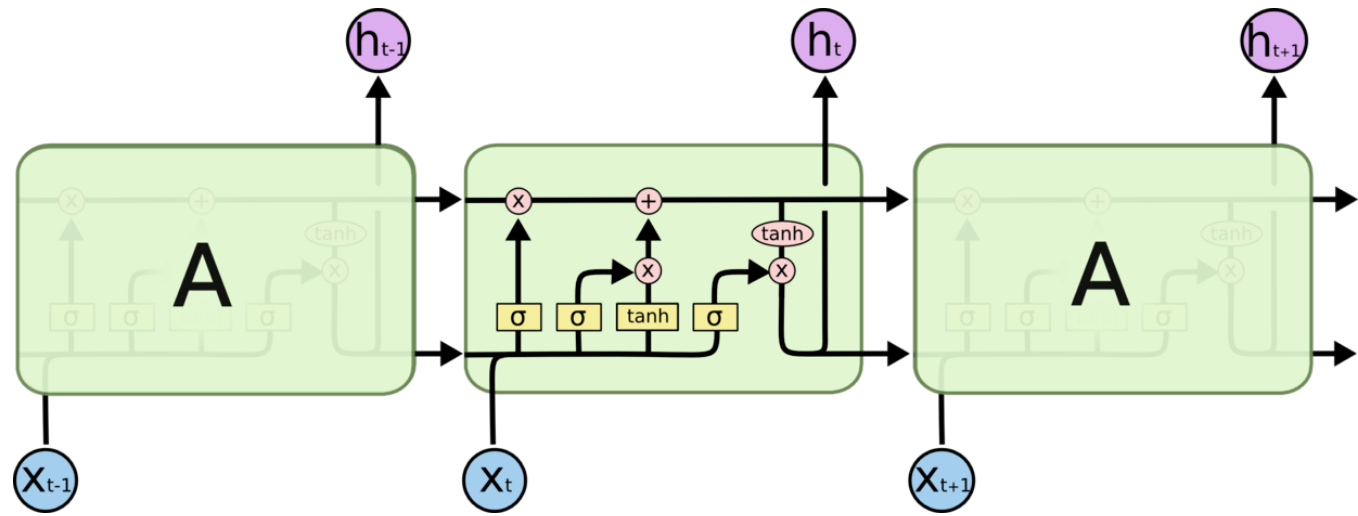输入输出结构
在n时刻
LSTM的输入有三个：
1.当前时刻网络的输入值Xt；
2.上一时刻LSTM的输出值ht-1；
3.上一时刻的单元状态Ct-1。
LSTM的输出有两个：
1.当前时刻LSTM输出值ht；
2.当前时刻的单元状态Ct。
门结构
门有三类：忘记门，输入门，输出门。
1.忘记门：以一定的概率控制是否遗忘上一层的隐藏细胞状态；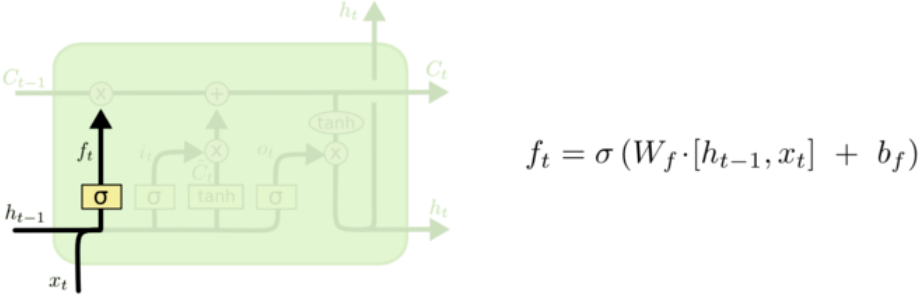2.输入门：负责处理当前序列位置的输入，更新细胞状态；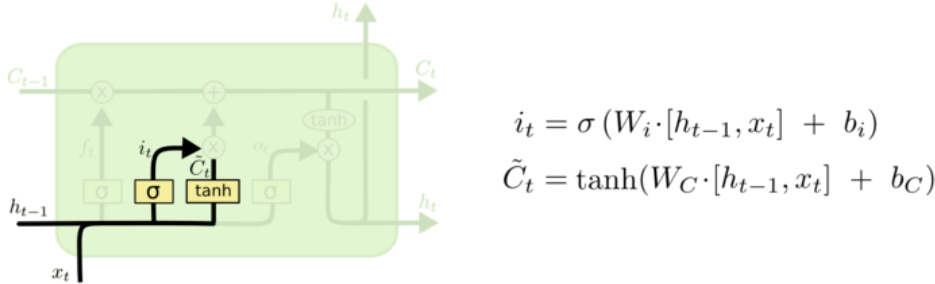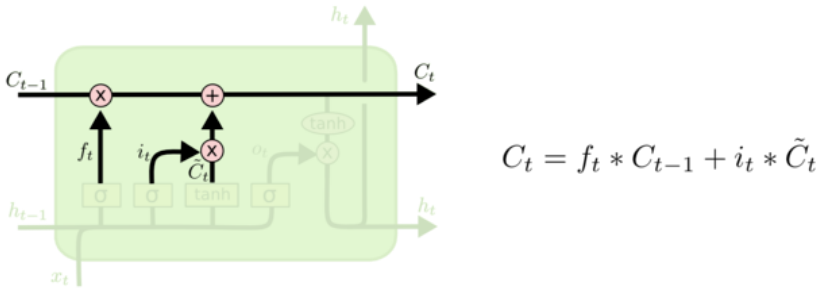3.输出门：决定输出什么。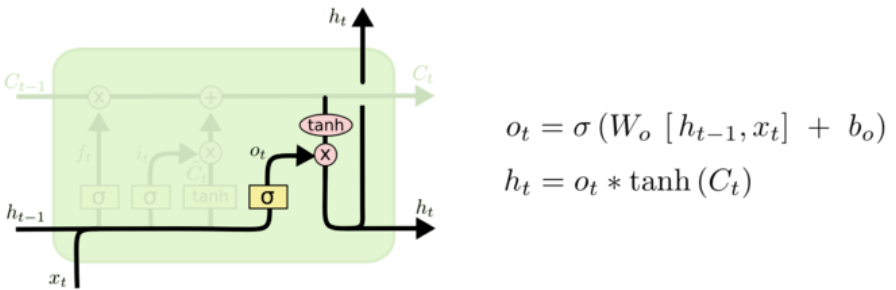参数计算
假设输入为：
TIME_STEPS = 28		# 时间步
INPUT_SIZE = 28		# 每个时间步的特征长度m
CELL_SIZE = 100		# 隐藏神经元个数n
OUTPUT_SIZE = 10	# 输出长度
inputs = Input(shape=[TIME_STEPS,INPUT_SIZE])

LSTM：
x = LSTM(CELL_SIZE, input_shape = (TIME_STEPS,INPUT_SIZE), return_sequences=False)(inputs)

输出：
x = Dense(OUTPUT_SIZE)(x)
x = Activation("softmax")(x)
model = Model(inputs,x)

网络参数：
Layer (type)                 Output Shape              Param #
=================================================================
input_1 (InputLayer)         (None, 28, 28)            0
_________________________________________________________________
lstm_1 (LSTM)                (None, 100)               51600
_________________________________________________________________
dense_1 (Dense)              (None, 10)                1010
_________________________________________________________________
activation_1 (Activation)    (None, 10)                0
=================================================================
Total params: 52,610
Trainable params: 52,610
Non-trainable params: 0

其中：
总参数 = LSTM层 + Dense层 = 51600 + 1010 = 52610
LSTM层：51600 = 4 x ((input_size+cell_size) x cell_size+cell_size) = 4x((28+100)x100+100)
Dense层：1010 = 100 x 10 + 10


展开全文• 可以首先计算LSTM的参数数量. 那么, 一个LSTM有多少个参数呢? 首先,文中提到LSTM在每层使用了1000个cell(对应于 与 的维度)与大小为1000的word embedding(最底层LSTM的输入大小等于word embedding的维度大小,其他层...
• 总体来说，假设lstm一个时间点上输入特征长度是n，输出长度是m，那么参数量是4*((n+m)*m+m)。 详细推导看这篇文章，讲得很详细很明白。
• 参数 – input_size – hidden_size – num_layers – bias – batch_first – dropout – bidirectional 输入 – input (seq_len, batch, input_size) – h_0 (num_layers * num_directions, batch, hidden_size) ...
• ## LSTM参数个数计算

万次阅读 多人点赞 2018-09-21 16:53:35
今天看程序看到了LSTM，原理都懂，但是想看看参数的个数是怎么...LSTM这一层的参数个数是 1255424 个，这一看还有整有零的，好奇怎么算出来的，于是百度搜了下，得到这么一个公式： 设 LSTM 输入维度为 x_dim， 输...
• ## cnn、lstm参数计算

千次阅读 2018-12-17 10:27:54
一、cnn参数计算   假设输入7*7*3，卷积核3*3*2，stride=(1,1),输出是3*3*2 参数量计算：fileter_size✖️input_channel✖️output_channel 解释:参数量与输入尺寸无关，只是卷积核的参数，每一个卷积核首先...
• LSTM的计算方式如下（不加偏置）： 可以看到若不加偏置，该LSTM层共有4个权重参数，图中输入的一个timestep的特征大小为3（绿色单元），隐藏层输出大小为2（橙色单元），因此该LSTM层的参数个数为： n=4∗(2+3)∗2=...
• 长期困惑与LSTM的神经元个数计算，记录一下当下的理解 A代表LSTM cell，同一个LSTM但是timestamp不同，由左图可知一个timestamp中有四个前馈神经元(黄色的，3个sigmoid和一个tanh) 图中还有一个tanh(红色的)，这里...深度学习 神经网络 tensorflow
• LSTM的参数训练和前向计算比RNNs还是稍微复杂一些。 长短时记忆网络的前向计算 前面描述的开关是怎样在算法中实现的呢？这就用到了门（gate）的概念。门实际上就是一层全连接层，它的输入是一个向量，输出是一...
• 1. 具体在看看几个门的位置和作用 lstm理解与使用(pytorch为例) 遗忘门的构成和参数 输入门 输出门 2. 参数量计算 如何计算 LSTM 的参数
• 关于LSTM的原理网上很多，被引用最多的应该就是这篇博客了Understanding LSTM Networks，它的译文也是遍布网上各个角落 使用LSTM分类mnist数据集的数字，数字图片大小28∗2828∗2828*28，每一步输入一行数据，总共...keras 深度学习
• 例如，设置’ ‘ num_layers=2 ‘ ‘意味着将两个LSTM堆叠在一起，形成一个’堆叠的LSTM ‘，第二个LSTM接收第一个LSTM的输出并计算最终结果。默认值:1 bias:如果’ False’，则该层不使用偏置权重’ b_ih ‘和’ b_...
• 神经网络学习小记录36——Keras实现LSTM学习前言什么是LSTM1、LSTM的结构2、LSTM独特的门结构3、LSTM参数计算a、遗忘门b、输入门c、输出门d、全部参数量在Keras中实现LSTM实现代码 学习前言 我死了我死了我死了！ ...Keras 神经网络 深度学习
• 如果对于参数计算过程不理解，那么对网络可能也并不是很理解。如图 上图是一个简单RNN示意图，为了方便理解，都会画成下面形式 这样就会造成一些误解，将RNN理解为t个不同模块，并且错误认为每个...
• 先回忆一下LSTM，直接上pytorch官网公式截图： 它可以处理变长序列，每个rnn cell参数是一样，共享，就是上面列出...BiLSTM包含一个从左到右和一个从右到左并列的的序列计算。需要注意是，两个方向使用...
• 对绝缘栅双极型晶体管参数进行分析，设计了一个基于LSTM网络绝缘栅双极型晶体管参数预测SoC硬件系统。该系统使用ARM处理器作为总控制器，控制各个子模块调用和数据传输，FPGA内通过对矩阵向量内积算法进行优化...长短期记忆网络
• 记录下自己LSTM这几天卡住我的地方 1：LSTM的输入由上一个时间的细胞输出h(t-1)与这一个时间...3：一些tensorflow中LSTM的参数理解，input_timesteps表示这一个环节拥有多少个lstm的小系统，比如我现在有32492的数据，深度学习 人工智能 tensorflow
• LSTM的参数计算 一. LSTM简介 LSTM：即Long Short-tem Memory，长短期记忆神经网络，1997年就被提出来了。 传统RNN的缺点，只能保留前一时刻的t-1的memory；而LSTM通过增加了3个门（输入门，遗忘门，输出门），来...深度学习 nlp
• LSTM参数计算 keras中若干个Cell例如LSTMCell 1.RNN循环神经网络 先来解释一下 batch_size timestep 样本数据： 小明爱学习 小王爱学习 小李爱学习 小花爱学习 通常样本数据会以(batch_size, time_step, ...神经网络 深度学习 算法 rnn
• NER中的LSTM+CRF2.1 CRF特征定义2.2 参数估计2.3 全局正则项的计算优化参考文献 在用LSTM+CRF做命名实体识别任务时，由于pytorch框架crf需要自己实现，网上很多教程都跳过了一些关键部分导致自己难以理解。......